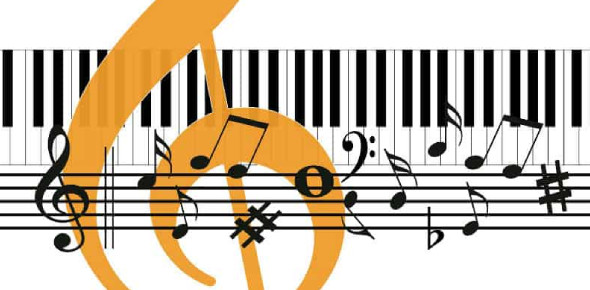# Quiz: Can You Pass The Music Theory Exam?

17 Questions | Total Attempts: 9425SettingsIn music, there is the practical part which is very broad and involves use of instruments and sound in creation but prior to that there is the theory part, which is essential for every student. All the best.

• 1.
A ____________________ is drawn as an open oval.
• A.

Half Note

• B.

Quarter Note

• C.

Half Rest

• D.

Whole Note

• 2.
A ____________________ means to rest (or remain silent) for an entire measure.
• A.

Whole Note

• B.

Whole Rest

• C.

Half Note

• D.

Half Rest

• 3.
Four __________________________ equal the duration of one whole note.
• A.

Quarter Notes

• B.

Half Notes

• C.

Eighth Notes

• D.

Whole Notes

• 4.
A __________________ is equal to half of a whole rest and sits on the 3rd line.
• A.

Whole Rest

• B.

Half Rest

• C.

Quarter Rest

• D.

Eighth Rest

• 5.
A ________________________ is equal to one quarter of a whole rest.
• A.

Quarter Rest

• B.

Half Note

• C.

Whole Note

• D.

Half Rest

• 6.
Two ____________________ = Four Quarter Notes
• A.

Measures

• B.

Quarter Notes

• C.

Whole Notes

• D.

Half Notes

• 7.
Four __________________________ = Two Half Rests
• A.

Quarter Rests

• B.

Half Rests

• C.

Measures

• D.

Whole Notes

• 8.
Two __________________________ = One Half Note
• A.

Bars

• B.

Quarter Notes

• C.

Measures

• D.

Clefs

• 9.
What 7 letters of the alphabet are used in music? __________________________
• A.

The First Seven A,B,C,D,E,F,G

• B.

E,G,B,D, AND F

• C.

F,A,C, AND E

• D.

The Last Seven A,B,C,D,E,F,G

• 10.
How many spaces are on a single staff? ________
• A.

4

• B.

3

• C.

2

• D.

1

• 11.
Two ____________________ equal the duration of one whole note.
• A.

Half notes

• B.

Quarter notes

• C.

Whole notes

• D.

Whole rest

• 12.
A Whole Note/Rest =________Beats
• A.

1

• B.

2

• C.

4

• D.

5

• 13.
A Half Note/Rest =________Beats
• A.

1

• B.

2

• C.

4

• D.

5

• 14.
A Quarter Note/Rest =________Beat
• A.

1

• B.

2

• C.

4

• D.

5

• 15.
What Clef is also known as the F Clef?
• A.

Treble Clef

• B.

Bass Clef

• 16.
What Clef is also known as the G Clef?
• A.

Treble Clef

• B.

Bass Clef

• 17.
Examining a song in Music Class is an example of . . .
• A.

Casual Listening

• B.

Sensual Listening

• C.

Perceptive Listening

Related TopicsBack to top